## Example 37.3 Enforcing Homogeneity Constraints: Count and Dispersion—It Is All Over!

The following example demonstrates how you can use either the EQUATE= option in the MODEL statement or the RESTRICT statement to impose homogeneity constraints on chosen model effects.

The data for this example were presented by Margolin, Kaplan, and Zeiger (1981) and analyzed by various authors applying a number of techniques. The following DATA step shows the number of revertant salmonella colonies (variable num) at six levels of quinoline dosing (variable dose). There are three replicate plates at each dose of quinoline.

```data assay;
label dose = 'Dose of quinoline (microg/plate)'
num  = 'Observed number of colonies';
input dose @;
logd = log(dose+10);
do i=1 to 3; input num@; output; end;
datalines;
0  15 21 29
10  16 18 21
33  16 26 33
100  27 41 60
333  33 38 41
1000  20 27 42
;
```

The basic notion is that the data are overdispersed relative to a Poisson distribution in which the logarithm of the mean count is modeled as a linear regression in dose (in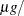plate) and in the derived variable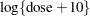(Lawless 1987). The log of the expected count of revertants is thus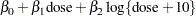The following statements fit a standard Poisson regression model to these data:

```proc fmm data=assay;
model num = dose logd / dist=Poisson;
run;
```

The Pearson statistic for this model is rather large compared to the number of degrees of freedom (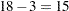). The ratio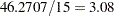indicates an overdispersion problem in the Poisson model (Output 37.3.1).

Output 37.3.1 Result of Fitting Poisson Regression Models
The FMM Procedure

 Number of Observations Read 18 18

Fit Statistics
-2 Log Likelihood 136.3
AIC (smaller is better) 142.3
AICC (smaller is better) 144.0
BIC (smaller is better) 144.9
Pearson Statistic 46.2707

Parameter Estimates for 'Poisson' Model
Effect Estimate Standard Error z Value Pr > |z|
Intercept 2.1728 0.2184 9.95 <.0001
dose -0.00101 0.000245 -4.13 <.0001
logd 0.3198 0.05700 5.61 <.0001

Breslow (1984) accounts for overdispersion by including a random effect in the predictor for the log rate and applying a quasi-likelihood technique to estimate the parameters. Wang et al. (1996) examine these data using mixtures of Poisson regression models. They fit several two- and three-component Poisson regression mixtures. Examining the log likelihoods, AIC, and BIC criteria, they eventually settle on a two-component model in which the intercepts vary by category and the regression coefficients are the same. This mixture model can be written as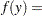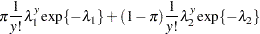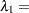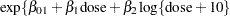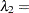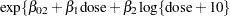This model is fit with the FMM procedure with the following statements:

```proc fmm data=assay;
model num = dose logd / dist=Poisson k=2
equate=effects(dose logd);
run;
```

The EQUATE= option in the MODEL statement places constraints on the optimization and makes the coefficients for dose and logd homogeneous across components in the model. Output 37.3.2 displays the "Fit Statistics" and parameter estimates in the mixture. The Pearson statistic is drastically reduced compared to the Poisson regression model in Output 37.3.1. With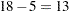degrees of freedom, the ratio of the Pearson and the degrees of freedom is now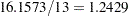. Note that the effective number of parameters was used to compute the degrees of freedom, not the total number of parameters, because of the equality constraints.

Output 37.3.2 Result for Two-Component Poisson Regression Mixture
The FMM Procedure

Fit Statistics
-2 Log Likelihood 121.8
AIC (smaller is better) 131.8
AICC (smaller is better) 136.8
BIC (smaller is better) 136.3
Pearson Statistic 16.1573
Effective Parameters 5
Effective Components 2

Parameter Estimates for 'Poisson' Model
Component Effect Estimate Standard Error z Value Pr > |z|
1 Intercept 1.9097 0.2654 7.20 <.0001
1 dose -0.00126 0.000273 -4.62 <.0001
1 logd 0.3639 0.06602 5.51 <.0001
2 Intercept 2.4770 0.2731 9.07 <.0001
2 dose -0.00126 0.000273 -4.62 <.0001
2 logd 0.3639 0.06602 5.51 <.0001

Parameter Estimates for Mixing Probabilities
Estimate Standard Error z Value Pr > |z|
Intercept 1.4984 0.6875 2.18 0.0293 0.8173

You could also have used RESTRICT statements to impose the homogeneity constraints on the model fit, as shown in the following statements:

```proc fmm data=assay;
model num = dose logd / dist=Poisson k=2;
restrict 'common dose' dose 1, dose -1;
restrict 'common logd' logd 1, logd -1;
run;
```

The first RESTRICT statement equates the coefficients for the dose variable in the two components, and the second RESTRICT statement accomplishes the same for the coefficients of the logd variable. If the right-hand side of a restriction is not specified, PROC FMM defaults to equating the left-hand side of the restriction to zero. The "Linear Constraints" table in Output 37.3.3 shows that both linear equality constraints are active. The parameter estimates match the previous FMM run.

Output 37.3.3 Result for Two-Component Mixture with RESTRICT Statements
The FMM Procedure

Linear Constraints at Solution
Label k = 1 k = 2   Constraint
Active
common dose dose - dose = 0 Yes
common logd logd - logd = 0 Yes

Parameter Estimates for 'Poisson' Model
Component Effect Estimate Standard Error z Value Pr > |z|
1 Intercept 1.9097 0.2654 7.20 <.0001
1 dose -0.00126 0.000273 -4.62 <.0001
1 logd 0.3639 0.06602 5.51 <.0001
2 Intercept 2.4770 0.2731 9.07 <.0001
2 dose -0.00126 0.000273 -4.62 <.0001
2 logd 0.3639 0.06602 5.51 <.0001

Parameter Estimates for Mixing Probabilities
Estimate Standard Error z Value Pr > |z|
Intercept 1.4984 0.6875 2.18 0.0293 0.8173

Wang et al. (1996) note that observation 12 with a revertant colony count of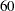is comparably high. The following statements remove the observation from the analysis and fit their selected model:

```proc fmm data=assay(where=(num ne 60));
model num = dose logd / dist=Poisson k=2
equate=effects(dose logd);
run;
```

Output 37.3.4 Result for Two-Component Model without Outlier
The FMM Procedure

Fit Statistics
-2 Log Likelihood 111.5
AIC (smaller is better) 121.5
AICC (smaller is better) 126.9
BIC (smaller is better) 125.6
Pearson Statistic 16.5987
Effective Parameters 5
Effective Components 2

Parameter Estimates for 'Poisson' Model
Component Effect Estimate Standard Error z Value Pr > |z|
1 Intercept 2.2272 0.3022 7.37 <.0001
1 dose -0.00065 0.000445 -1.46 0.1440
1 logd 0.2432 0.1045 2.33 0.0199
2 Intercept 2.5477 0.3331 7.65 <.0001
2 dose -0.00065 0.000445 -1.46 0.1440
2 logd 0.2432 0.1045 2.33 0.0199

Parameter Estimates for Mixing Probabilities
Estimate Standard Error z Value Pr > |z|
Intercept 0.3134 1.7261 0.18 0.8559 0.5777

The ratio of Pearson Statistic over degrees of freedom (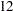) is only slightly worse than in the previous model; the loss of 5% of the observations carries a price (Output 37.3.4). The parameter estimates for the two intercepts are now fairly close. If the intercepts were identical, then the two-component model would collapse to the Poisson regression model:

```proc fmm data=assay(where=(num ne 60));
model num = dose logd / dist=Poisson;
run;
```

Output 37.3.5 Result of Fitting Poisson Regression Model without Outler
The FMM Procedure

 Number of Observations Read 17 17

Fit Statistics
-2 Log Likelihood 114.1
AIC (smaller is better) 120.1
AICC (smaller is better) 121.9
BIC (smaller is better) 122.5
Pearson Statistic 27.8008

Parameter Estimates for 'Poisson' Model
Effect Estimate Standard Error z Value Pr > |z|
Intercept 2.3164 0.2244 10.32 <.0001
dose -0.00072 0.000258 -2.78 0.0055
logd 0.2603 0.05996 4.34 <.0001

Compared to the same model applied to the full data, the Pearson statistic is much reduced (compare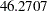in Output 37.3.1 to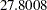in Output 37.3.5). The outlier—or overcount, if you will—induces at least some of the overdispersion.